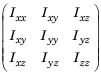## Robot Dynamics

This topic details the different elements, properties, and equations of rigid body robot dynamics. Robot dynamics are the relationship between the forces acting on a robot and the resulting motion of the robot. In Robotics System Toolbox™, manipulator dynamics information is contained within a `rigidBodyTree` object, which specifies the rigid bodies, attachment points, and inertial parameters for both kinematics and dynamics calculations.

Note

To use dynamics object functions, you must set the `DataFormat` property of the `rigidBodyTree` object to `"row"` or `"column"`. These setting accept inputs and return outputs as row or column vectors, respectively, for relevant robotics calculations, such as robot configurations or joint torques.

### Dynamics Properties

When working with robot dynamics, specify the information for individual bodies of your manipulator robot using these properties of the `rigidBody` objects:

• `Mass` — Mass of the rigid body in kilograms.

• `CenterOfMass` — Center of mass position of the rigid body, specified as a vector of the form `[x y z]`. The vector describes the location of the center of mass of the rigid body, relative to the body frame, in meters. The `centerOfMass` object function uses these rigid body property values when computing the center of mass of a robot.

• `Inertia` — Inertia of the rigid body, specified as a vector of the form ```[Ixx Iyy Izz Iyz Ixz Ixy]```. The vector is relative to the body frame in kilogram square meters. The inertia tensor is a positive definite matrix of the form:The first three elements of the `Inertia` vector are the moment of inertia, which are the diagonal elements of the inertia tensor. The last three elements are the product of inertia, which are the off-diagonal elements of the inertia tensor.

For information related to the entire manipulator robot model, specify these `rigidBodyTree` object properties:

• `Gravity` — Gravitational acceleration experienced by the robot, specified as an ```[x y z]``` vector in m/s2. By default, there is no gravitational acceleration.

• `DataFormat` — The input and output data format for the kinematics and dynamics functions, specified as `"struct"`, `"row"`, or `"column"`.

### Dynamics Equations

Manipulator rigid body dynamics are governed by this equation:

`$\frac{d}{dt}\left[\begin{array}{c}q\\ \stackrel{˙}{q}\end{array}\right]=\left[\begin{array}{c}\stackrel{˙}{q}\\ M{\left(q\right)}^{-1}\left(-C\left(q,\stackrel{˙}{q}\right)\stackrel{˙}{q}-G\left(q\right)-J{\left(q\right)}^{T}{F}_{Ext}+\tau \right)\end{array}\right]$`

also written as:

`$M\left(q\right)\stackrel{¨}{q}=-C\left(q,\stackrel{˙}{q}\right)\stackrel{˙}{q}-G\left(q\right)-J{\left(q\right)}^{T}{F}_{Ext}+\tau$`

where:

• $M\left(q\right)$ — is a joint-space mass matrix based on the current robot configuration. Calculate this matrix by using the `massMatrix` object function.

• $C\left(q,\stackrel{˙}{q}\right)$ — are the Coriolis terms, which are multiplied by $\stackrel{˙}{q}$ to calculate the velocity product. Calculate the velocity product by using by the `velocityProduct` object function.

• $G\left(q\right)$ — is the gravity torques and forces required for all joints to maintain their positions in the specified gravity `Gravity`. Calculate the gravity torque by using the `gravityTorque` object function.

• $J\left(q\right)$ — is the geometric Jacobian for the specified joint configuration. Calculate the geometric Jacobian by using the `geometricJacobian` object function.

• ${F}_{Ext}$ — is a matrix of the external forces applied to the rigid body. Generate external forces by using the `externalForce` object function.

• $\tau$ — are the joint torques and forces applied directly as a vector to each joint.

• $q,\stackrel{˙}{q},\stackrel{¨}{q}$ — are the joint configuration, joint velocities, and joint accelerations, respectively, as individual vectors. For revolute joints, specify values in radians, rad/s, and rad/s2, respectively. For prismatic joints, specify in meters, m/s, and m/s2.

To compute the dynamics directly, use the `forwardDynamics` object function. The function calculates the joint accelerations for the specified combinations of the above inputs.

To achieve a certain set of motions, use the `inverseDynamics` object function. The function calculates the joint torques required to achieve the specified configuration, velocities, accelerations, and external forces.